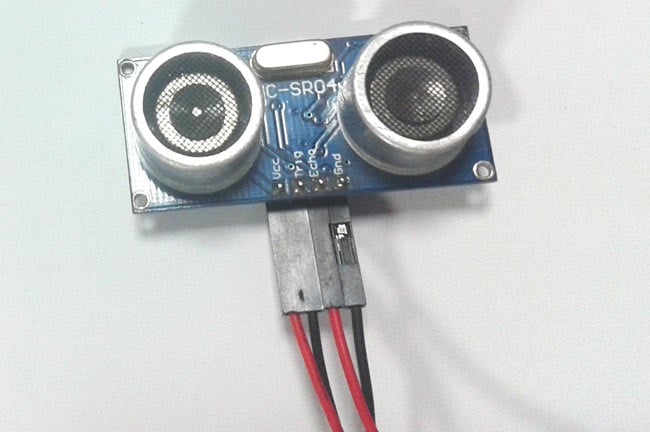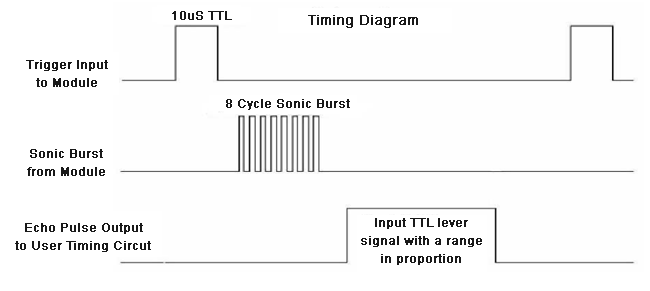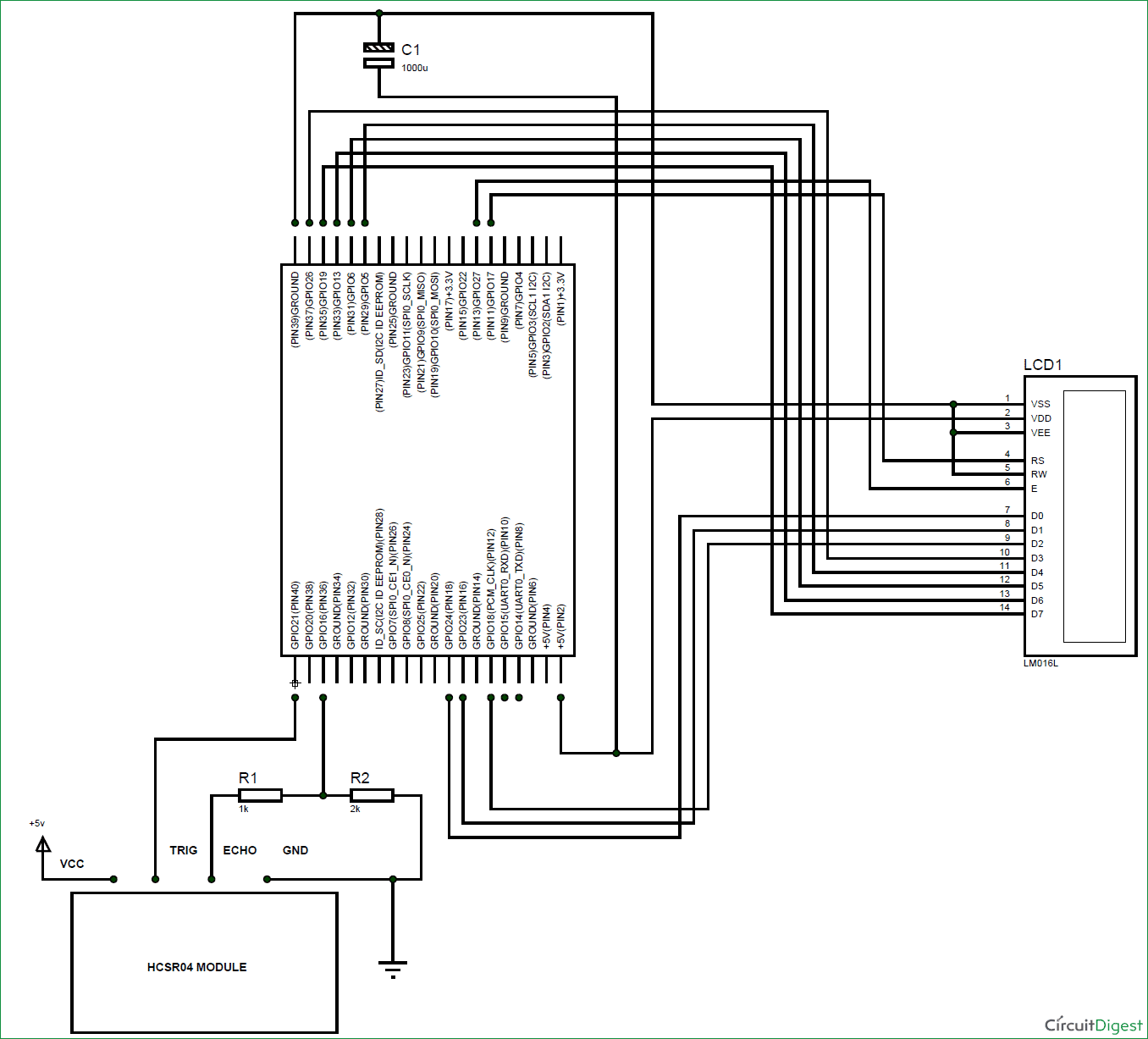In this project we are going to interface HC-SR04 Ultrasonic sensor module to Raspberry Pi to measure distance. We have previously used Ultrasonic sensor with Raspberry Pi to build Obstacle Avoiding Robot. Before going any further, lets know about Ultrasonic sensor.

### HC-SR04 Ultrasonic Sensor:

The Ultrasonic Sensor is used to measure the distance with high accuracy and stable readings. It can measure distance from 2cm to 400cm or from 1 inch to 13 feet.  It emits an ultrasound wave at the frequency of 40KHz in the air and if the object will come in its way then it will bounce back to the sensor. By using that time which it takes to strike the object and comes back, you can calculate the distance.The ultrasonic sensor uses a technique called “ECHO”. “ECHO” is simply a reflected sound wave. You will have an ECHO when sound reflects back after reaching a dead end.

HCSR04 module generates a sound vibration in ultrasonic range when we make the ‘Trigger’ pin high for about 10us which will send a 8 cycle sonic burst at the speed of sound and after striking the object, it will be received by the Echo pin. Depending on time taken by sound vibration to get back, it provides appropriate pulse output. If the object is far away then it takes more time for ECHO to be heard and the output pulse width will be big. And if the obstacle is near, then the ECHO will be heard faster and output pulse width will be smaller.We can calculate the distance of the object based on the time taken by ultrasonic wave to return back to the sensor. Since the time and speed of sound is known we can calculate the distance by the following formulae.

• Distance= (Time x Speed of Sound in Air (343 m/s))/2.

The value is divided by two since the wave travels forward and backward covering the same distance.Thus the time to reach obstacle is just half the total time taken

So Distance in centimeter = 17150*T

We have previously made many useful project using this Ultrasonic sensor and Arduino, check them below:

### Components Required:

Here we are using Raspberry Pi 2 Model B with Raspbian Jessie OS. All the basic Hardware and Software requirements are previously discussed, you can look it up in the Raspberry Pi Introduction and Raspberry PI LED Blinking for getting started, other than that we need:

• Raspberry Pi with pre-installed OS
• HC-SR04 Ultrasonic Sensor
• Power supply (5v)
• 1KΩ resistor (3 pieces)
• 1000uF capacitor
• 16*2 character LCD

### Circuit Explanation:

Connections between Raspberry Pi and LCD are given in the below table:

 LCD connection Raspberry Pi connection GND GND VCC +5V VEE GND RS GPIO17 R/W GND EN GPIO27 D0 GPIO24 D1 GPIO23 D2 GPIO18 D3 GPIO26 D4 GPIO5 D5 GPIO6 D6 GPIO13 D7 GPIO19

In this circuit, we used 8bit communication (D0-D7) to connect LCD with Raspberry Pi, however this is not a compulsory, we can also use 4-bit communication (D4-D7), but with 4 bit communication program becomes a bit complex for beginners so just go with 8 bit communication. Here we have connected 10 pins of LCD to Raspberry Pi in which 8 pins are data pins and 2 pins are control Pins.

Below is the circuit diagram for connecting HC-SR04 sensor and LCD with Raspberry Pi for measuring the distance.As shown in the figure, HC-SR04 Ultrasonic Sensor has four pins,

1. PIN1- VCC or +5V
2. PIN2- TRIGGER (10us High pulse given to tell the sensor to sense the distance)
3. PIN3- ECHO (Provides pulse output whose width represents distance after trigger)
4. PIN4- GROUND

Echo pin provides +5V output pulse which cannot be connected to Raspberry Pi directly. So we will be using Voltage Divider Circuit (built using R1 and R2) to get +3.3V logic instead of +5V logic.

### Working Explanation:

Complete working of Raspberry Pi Distance Measure goes as,

1. Triggering the sensor by pulling up the trigger pin for 10uS.

2. Sound wave is sent by the sensor. After receiving the ECHO, sensor module provides an output proportional to distance.

3. We will record the time when the output pulse goes from LOW to HIGH and when again when its goes form HIGH to LOW.

4. We will have start and stop time. We will use distance equation to calculate the distance.

5. The distance is displayed in 16x2 LCD display.

Accordingly we have written the Python Program for Raspberry Pi to do the following functions:

1. To send trigger to sensor

2. Record start and stop time of pulse output from sensor.

3. To Calculate the distance by using START and STOP time.

4. To Display the result obtained on the 16*2 LCD.

Complete Program and Demo Video are given below. Program is well explained through the comments, if you have any doubt you can ask in comment section below.

Code

import time
import RPi.GPIO as IO    #calling for header file which helps in using GPIOs of PI

string_of_characters = 0

IO.setwarnings(False)    #do not show any warnings
IO.setmode (IO.BCM)      #programming the GPIO by BCM pin numbers. (like PIN29 as GPIO5)
IO.setup(17,IO.OUT)      #initialize GPIO17,27,24,23,18,26,5,6,13,19,21 as an output
IO.setup(27,IO.OUT)
IO.setup(24,IO.OUT)
IO.setup(23,IO.OUT)
IO.setup(18,IO.OUT)
IO.setup(26,IO.OUT)
IO.setup(5,IO.OUT)
IO.setup(6,IO.OUT)
IO.setup(13,IO.OUT)
IO.setup(19,IO.OUT)
IO.setup(21,IO.OUT)
IO.setup(16,IO.IN)      #initialize GPIO16 as an input

def send_a_command (command):  #steps for sending a command to 16x2 LCD
pin=command
PORT(pin);
IO.output(17,0)
#PORTD&= ~(1<<RS);
IO.output(27,1)
#PORTD|= (1<<E);
time.sleep(0.001)
#_delay_ms(50);
IO.output(27,0)
#PORTD&= ~(1<<E);
pin=0
PORT(pin);

def send_a_character (character):  #steps for sending a character to 16x2 LCD
pin=character
PORT(pin);
IO.output(17,1)
#PORTD|= (1<<RS);
IO.output(27,1)
#PORTD|= (1<<E);
time.sleep(0.001)
#_delay_ms(50);
IO.output(27,0)
#PORTD&= ~(1<<E);
pin=0
PORT(pin);

def PORT(pin):                    #assigning level for PI GPIO for sending data to LCD through D0-D7
if(pin&0x01 == 0x01):
IO.output(24,1)
else:
IO.output(24,0)
if(pin&0x02 == 0x02):
IO.output(23,1)
else:
IO.output(23,0)
if(pin&0x04 == 0x04):
IO.output(18,1)
else:
IO.output(18,0)
if(pin&0x08 == 0x08):
IO.output(26,1)
else:
IO.output(26,0)
if(pin&0x10 == 0x10):
IO.output(5,1)
else:
IO.output(5,0)
if(pin&0x20 == 0x20):
IO.output(6,1)
else:
IO.output(6,0)
if(pin&0x40 == 0x40):
IO.output(13,1)
else:
IO.output(13,0)
if(pin&0x80 == 0x80):
IO.output(19,1)
else:
IO.output(19,0)

def send_a_string(string_of_characters):
string_of_characters = string_of_characters.ljust(16," ")
for i in range(16):
send_a_character(ord(string_of_characters[i]))  #send characters one by one through data port

while 1:
send_a_command(0x38);  #16x2 line LCD
send_a_command(0x0E);  #screen and cursor ON
send_a_command(0x01);  #clear screen
time.sleep(0.1)                #sleep for 100msec

IO.setup(21,1)
time.sleep(0.00001)
IO.setup(21,0)           #sending trigger pulse for sensor to measure the distance

while (IO.input(16)==0):
start = time.time()  #store the start time of pulse output

while (IO.input(16)==1):
stop = time.time()   #store the stop time

distance = ((stop - start)*17150)  #calculate distance from time
distance = round(distance,2)       #round up the decimal values
if(distance<400):                  #if distance is less than 400 cm, display the result on LCD
send_a_command(0x80 + 0);
send_a_string ("Dist=%s cm"% (distance));
time.sleep(0.15)

if(distance>400):                  #If distance is more than 400cm, just print 400+ on LCD
send_a_command(0x80 + 0);
send_a_string ("Dist= 400+ cm");
time.sleep(0.15)

Video

Subscribe below to receive most popular news, articles and DIY projects from Circuit Digest

•May 01, 2018

Starting at about line 20 of the program - I see about 8 lines that start with the hashtag (#). My understanding of the Python language, hashtags are used for comments only and Python does not read these as commands or instructions.

• AISHA
May 03, 2018

Yes you are correct "#" in python is for comment lines

•Sridevi
Jul 19, 2018

Hi
First of all thanks for great explanation.
I'm running same code with same connections but not able to output on display.